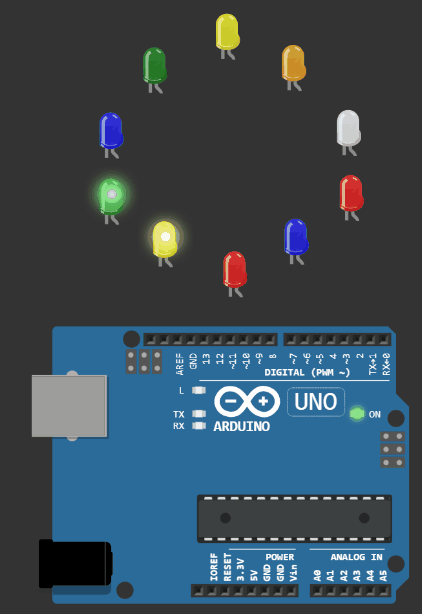The LED chaser circuit based on Arduino UNO. Complete code and project are available.

Short Introduction

LED chaser is always a favourite example for beginners because of the joy of looking at the LEDs dance 🕺. don't you agree?  You can find the code for the LED chaser as well as the connection diagrams needed. The simulations from Wokwi Arduino Simulator is used.LED chaser Circuit

```        ```//www.youtube.com/Electronics is Fun//
//Project by MOHD SOHAIL//

int pinsCount=10;                        // declaring the integer variable pinsCount
int pins[] = {2,3,4,5,6,7,8,9,10,11};          // declaring the array pins[]

void setup() {
for (int i=0; i<pinsCount; i=i+1){    // counting the variable i from 0 to 9
pinMode(pins[i], OUTPUT);            // initialising the pin at index i of the array of pins as OUTPUT
}
}

void loop() {
for (int i=0; i<pinsCount; i=i+1){    // chasing right
digitalWrite(pins[i], HIGH);         // switching the LED at index i on
delay(100);                          // stopping the program for 100 milliseconds
digitalWrite(pins[i], LOW);          // switching the LED at index i off
}
for (int i=pinsCount-1; i>0; i=i-1){   // chasing left (except the outer leds)
digitalWrite(pins[i], HIGH);         // switching the LED at index i on
delay(100);                          // stopping the program for 100 milliseconds
digitalWrite(pins[i], LOW);          // switching the LED at index i off
}
}```
```